# Python金融大数据分析-蒙特卡洛仿真

无意中发现了一个巨牛的人工智能教程，忍不住分享一下给大家。教程不仅是零基础，通俗易懂，而且非常风趣幽默，像看小说一样！觉得太牛了，所以分享给大家。教程链接：https://www.cbedai.net/qtlyx

## 1.简单的例子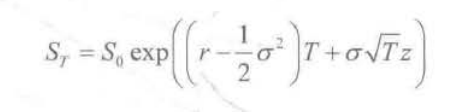了解一点金融工程的对这个公式都不会太陌生，是用现在股价预测T时间股价的公式，其背后是股价符合几何布朗运动，也就是大名鼎鼎的BSM期权定价模型的基础。

我们假设现在一个股票的价值是100，那么两年后是多少呢？

import numpy as np
import pandas as pd
import matplotlib.pyplot as plt
S0 = 100
r = 0.05
sigma = 0.25
T = 2.0
I = 10000
ST1 = S0*np.exp((r - 0.5*sigma**2)*T+sigma*np.sqrt(T)*np.random.standard_normal(I))
plt.hist(ST1,bins = 50)
plt.xlabel('price')
plt.ylabel('ferquency')

运行的结果如下所示：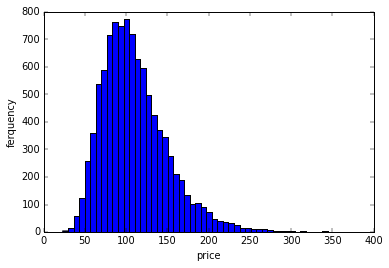很明显，是一个lognormal分布，因为这样的假设下，价格符合lognormal分布，收益率符合正态分布。

## 2.简单的蒙特卡洛路径

上面是一步到位的，那么如果我们中间分很多个小时间段来仿真呢？可以知道，物理问题是一样的，结果也不会有差异。

import numpy as np
import pandas as pd
import matplotlib.pyplot as plt
import scipy.stats as scs
S0 = 100
r = 0.05
sigma = 0.25
T = 2.0
I = 10000
#ST1 = S0*np.exp((r - 0.5*sigma**2)*T+sigma*np.sqrt(T)*np.random.standard_normal(I))
#plt.hist(ST1,bins = 50)
#plt.xlabel('price')
#plt.ylabel('ferquency')

M = 50
dt = T/M
S = np.zeros((M + 1,I))
S = S0
print S
for t in range(1,M+1):
S[t] = S[t-1]*np.exp((r-0.5*sigma**2)*dt+sigma*np.sqrt(dt)*np.random.standard_normal(I))
plt.hist(S[-1],bins = 50)
plt.xlabel('price')
plt.ylabel('frequency')
plt.show()
plt.plot(S[:,:],lw = 1.5)
plt.xlabel('time')
plt.ylabel('price')
plt.show()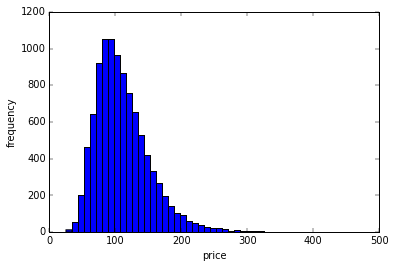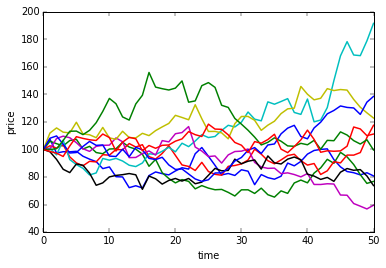我们不仅可以得到最终的分布，也可以知道价格路径，而这一价格路径，才是真正代表了蒙特卡洛的精髓。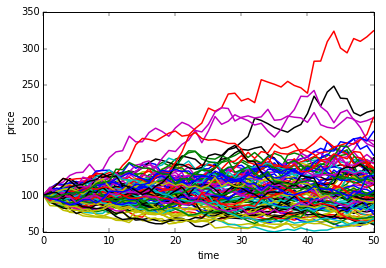从侧面看，其实就是一个lognormal分布。